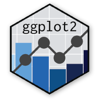Show Sidebar Hide Sidebar# geom_abline in ggplot2

How to use the abline geom in ggplot2 to add a line with specified slope and intercept to the plot.

### New to Plotly?

Plotly's R library is free and open source!
You can set up Plotly to work in online or offline mode.
We also have a quick-reference cheatsheet (new!) to help you get started!

### Version Check

Version 4 of Plotly's R package is now available!
Check out this post for more information on breaking changes and new features available in this version.

library(plotly)
packageVersion('plotly')

##  '4.9.1'


### Line

add line for mean using geom_vline

library(plotly)

set.seed(1234)
dat <- data.frame(cond = factor(rep(c("A","B"), each=200)),
rating = c(rnorm(200),rnorm(200, mean=.8)))

p <- ggplot(dat, aes(x=rating)) +
geom_histogram(binwidth=.5, colour="black", fill="white") +
geom_vline(aes(xintercept=mean(rating, na.rm=T)),   # Ignore NA values for mean
color="red", linetype="dashed", size=1)

p <- ggplotly(p)

p


### Histogram

overlaid histograms with geom_vline

library(plotly)
library(plyr)
cdat <- ddply(dat, "cond", summarise, rating.mean=mean(rating))

# Overlaid histograms with means
p <- ggplot(dat, aes(x=rating, fill=cond)) +
geom_histogram(binwidth=.5, alpha=.5, position="identity") +
geom_vline(data=cdat, aes(xintercept=rating.mean),
linetype="dashed", size=1)

p <- ggplotly(p)

p


### Histogram Means

histograms with geom_vline means

library(plotly)
library(plyr)
cdat <- ddply(dat, "cond", summarise, rating.mean=mean(rating))

# With mean lines
p <- ggplot(dat, aes(x=rating)) + geom_histogram(binwidth=.5, colour="black", fill="white") +
facet_grid(cond ~ .) +
geom_vline(data=cdat, aes(xintercept=rating.mean),
linetype="dashed", size=1, colour="red")

p <- ggplotly(p)

p


### Density Plots

density plots with geom_vline means

library(plotly)
library(plyr)
cdat <- ddply(dat, "cond", summarise, rating.mean=mean(rating))

# Density plots with means
p <- ggplot(dat, aes(x=rating, colour=cond)) +
geom_density() +
geom_vline(data=cdat, aes(xintercept=rating.mean),
linetype="dashed", size=1)

p <- ggplotly(p)

p


### Horizontal Line

add horizontal line with geom_hline

library(plotly)

cond xval yval
control 11.5 10.8
control  9.3 12.9
control  8.0  9.9
control 11.5 10.1
control  8.6  8.3
control  9.9  9.5
control  8.8  8.7
control 11.7 10.1
control  9.7  9.3
control  9.8 12.0
treatment 10.4 10.6
treatment 12.1  8.6
treatment 11.2 11.0
treatment 10.0  8.8
treatment 12.9  9.5
treatment  9.1 10.0
treatment 13.4  9.6
treatment 11.6  9.8
treatment 11.5  9.8
treatment 12.0 10.6
')

# The basic scatterplot
p <- ggplot(dat, aes(x=xval, y=yval, colour=cond)) +
geom_point()

p <- p + geom_hline(aes(yintercept=10))

p <- ggplotly(p)

p


### Mean Line

add mean line with geom_hline

library(plotly)

cond xval yval
control 11.5 10.8
control  9.3 12.9
control  8.0  9.9
control 11.5 10.1
control  8.6  8.3
control  9.9  9.5
control  8.8  8.7
control 11.7 10.1
control  9.7  9.3
control  9.8 12.0
treatment 10.4 10.6
treatment 12.1  8.6
treatment 11.2 11.0
treatment 10.0  8.8
treatment 12.9  9.5
treatment  9.1 10.0
treatment 13.4  9.6
treatment 11.6  9.8
treatment 11.5  9.8
treatment 12.0 10.6
')

# The basic scatterplot
p <- ggplot(dat, aes(x=xval, y=yval, colour=cond)) +
geom_point()

mean1 <- mean(dat[dat$cond == "control", "xval"]) mean2 <- mean(dat[dat$cond == "treatment", "xval"])

# Add colored lines for the mean xval of each group
p <- p +
geom_vline(aes(xintercept=mean1), colour="green") +
geom_vline(aes(xintercept=mean2), colour="lightblue")

p <- ggplotly(p)

p


### Geomvline & Geomhline

use geomvline with geomhline

library(plotly)

cond xval yval
control 11.5 10.8
control  9.3 12.9
control  8.0  9.9
control 11.5 10.1
control  8.6  8.3
control  9.9  9.5
control  8.8  8.7
control 11.7 10.1
control  9.7  9.3
control  9.8 12.0
treatment 10.4 10.6
treatment 12.1  8.6
treatment 11.2 11.0
treatment 10.0  8.8
treatment 12.9  9.5
treatment  9.1 10.0
treatment 13.4  9.6
treatment 11.6  9.8
treatment 11.5  9.8
treatment 12.0 10.6
')

# The basic scatterplot
p <- ggplot(dat, aes(x=xval, y=yval, colour=cond)) + geom_point()

# Add a red dashed vertical line
p <- p + geom_hline(aes(yintercept=10)) +
geom_vline(aes(xintercept=11.5), colour="#BB0000", linetype="dashed")

p <- ggplotly(p)

p


These ggplot2 examples were inspired by the Cookbook for R.